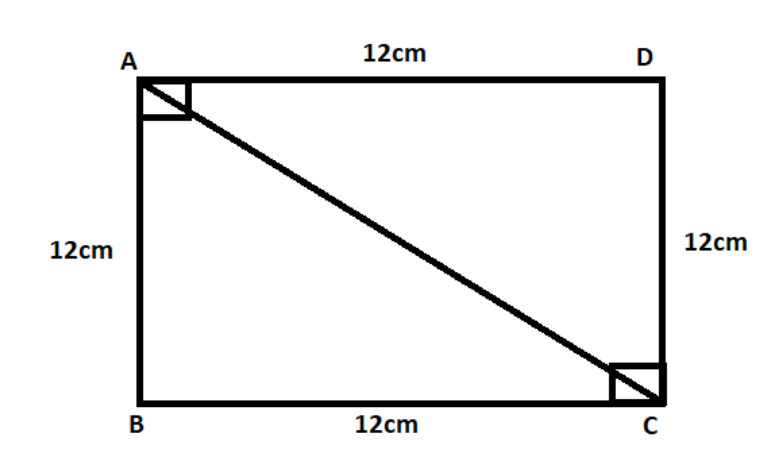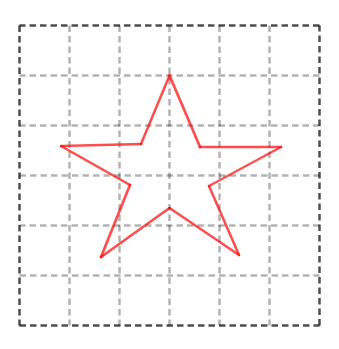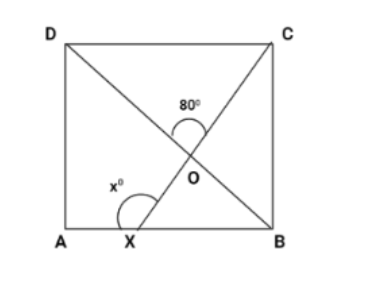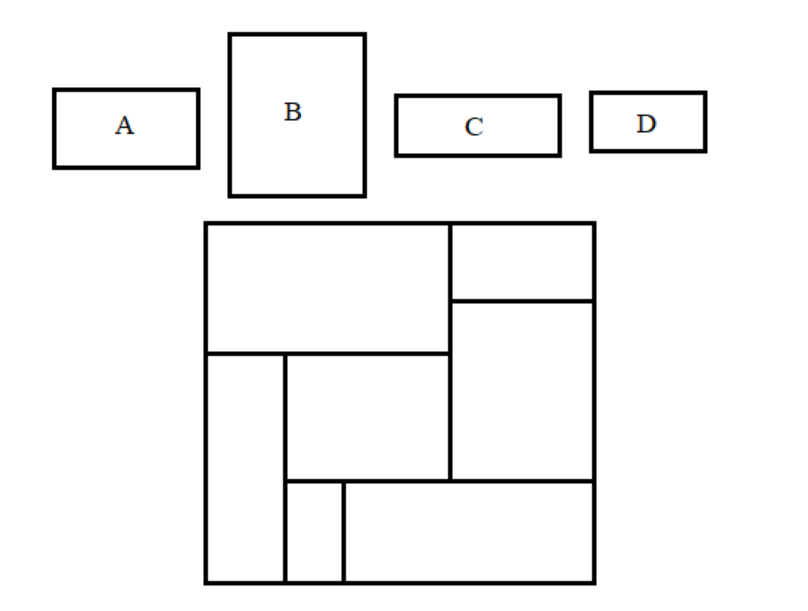Filters
Latest Questions
Mathematics
Square
At what angle diagonals of a square intersect each other ?

Mathematics
Square
Fill in the blank.
Two squares are congruent if ________.

Mathematics
Square
Diagonal of a square is $5\sqrt 2$ . Length of the side of the square is
$\left( A \right)$ $10$
$\left( B \right)$ $5$
$\left( C \right)$ $3\sqrt 2$
$\left( D \right)$ $2\sqrt 2$
Mathematics
Square
State true or false: All squares are not parallelograms.
A. true
B. false
C. ambiguous
D. data insufficient

Mathematics
Square
All squares are congruent.
State whether the above statement is true or false:
a). True
b). False
Mathematics
Square
Find the length of the diagonal of a square of side $12cm.$Mathematics
Square
Calculate area of the figure made by joining $25$ unit squares
1. $22\text{ }c{{m}^{2}}$
2. $23\text{ }c{{m}^{2}}$
3. $24\text{ }c{{m}^{2}}$
4. $25\text{ }c{{m}^{2}}$

Mathematics
Square
Calculate the area of irregular shape, if each square has side length of $1cm$. If the area is covering more than half of the square, then consider it as a full square.(A) Area is approximately $10sq.cm$
(B) Area is approximately $10sq.m$
(C) Area is approximately $6sq.cm$
(D) Area is approximately $6sq.m$
Mathematics
Square
In a square $\square ABCD$, the diagonals bisect each other at point $O$. What type of triangle is $\vartriangle AOB$?
A.An equilateral triangle
B.An isosceles but not right-angled triangle
C.A right-angled but not an isosceles triangle
D.An isosceles right-angled triangle

Mathematics
Square
In the adjoining figure, $ABCD$ is a square. A line segment $CX$ cuts $AB$ at $X$ and the diagonal $BD$ at $O$ such that $\angle COD = {80^o}$ and $\angle OXA = {x^o}$. Find the value of $x$.Mathematics
Square
Which rectangle A, B, C or D fits into the big square without turning?Mathematics
Square
The diagonals of a square are $\_\_\_\_\_$ .

Prev
1
2
3
4